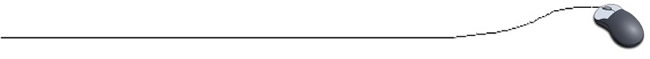Increment and Decrement Operators Adding or subtracting 1 from a variable is a very common programming practice.  Adding 1 to a variable is called incrementing and subtracting 1 from a variable is called decrementing. increment and decrement operators work only with integer variables -- not on floating point variables and not on literals. the execution of the prefix and postfix is under Java control -- nothing you can do will change the order of execution -- parentheses will not force an early execution:  num = (((x--)))* count;   //still decrements x last. a limitation with using the increment or decrement operator is that the variable being operated upon can only be incremented or decremented by the value ONE.  If you need to increment (or decrement) by a larger (or smaller) value, you must use statements like  j += 2;  or  value -= 5; be careful when combining increment and decrement operators inside expressions with logical operators.  If in doubt, don't use them together.  For example, do NOT use: if (( a = = 9 ) || ( b != ++k))  (k may not be incremented due to the short circuiting of the OR when (a = = 9) is TRUE). instead use: ++k; if (( a = = 9) || (b != k)) Be Careful!  i = i + 1  may look WRONG mathematically, but to a computer it means "take what is in variable i, add one to it, and put it back in variable i.  Computers always look on the right side first.  Remember, it is an assignment statement.All are the same: i = i + 1;  i += 1; i++;

 Prefix: ++i; --i; increment or decrement occurs before the variable's value is used in the remainder of the expression.

 Postfix: i++; i--; increment or decrement occurs after the variable's value is used in the remainder of the expression.

 Be careful when using increments within a print line. System.out.print(a++); a is incremented AFTER the printing occurs. System.out.print(++a); a is incremented BEFORE the printing occurs.